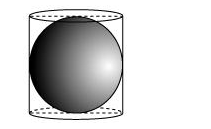# A right circular cylinder just encloses a sphere of radius r (see figure).

Question. A right circular cylinder just encloses a sphere of radius r (see figure). Find(i) surface area of the sphere,

(ii) curved surface area of the cylinder,

(iii) ratio of the areas obtained in (i) and (ii).

Solution:(i) Surface area of sphere $=4 \pi r^{2}$

(ii) Height of cylinder $=r+r=2 r$

Radius of cylinder $=r$

CSA of cylinder $=2 \pi r h$

$=2 \pi r(2 r)$

$=4 \pi r^{2}$

(iii) Required ratio $=\frac{\text { Surface area of sphere }}{\text { CSA of cylinder }}$

$=\frac{4 \pi r^{2}}{4 \pi r^{2}}$

$=\frac{1}{1}$

Therefore, the ratio between these two surface areas is $1: 1$.